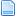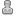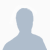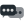Hello Guest it is October 22, 2019, 02:59:43 AMAuthor Topic: ATC Macro - How to Count Pulses?  (Read 2082 times)

0 Members and 1 Guest are viewing this topic.Chaz•48ATC Macro - How to Count Pulses?
« on: June 30, 2017, 08:12:00 AM »
So I have a my Denford using an IP-A. The toolchanger macro is basic but can be improved.

The Denford has either an NPN or PNP wired proximity sensor. The logic to work out which way to turn the ATC works but currently I use a 1 sec interval to assume 1 rev of the geared motor. It works but over time it gets out of sync. The best therefore is to count (or decrement) a number based on pulses. Any idea how I do that via a CS Labs input and VB?

ThanksTPS•1,389Re: ATC Macro - How to Count Pulses?
« Reply #1 on: July 02, 2017, 11:26:36 AM »
If ((GetInBit(90,0) = 1) and (GetOEMDro(2200) = 0) and (MotorturnCW=1)) then
SetOEMDro(2201) = GetOEMDro(2201) +1
End If

If ((GetInBit(90,0) = 1) and (GetOEMDro(2200) = 0) and (MotorturnCCW=1)) then
SetOEMDro(2201) = GetOEMDro(2201) -1
End If

If (GetInBit(90,0) = 1)  then
SetOEMDro(2200) = 1
Else
SetOEMDro(2200) = 0
End If

something like this should work, not tested just written down,
but i think you have to find a criteria to "reference" OemDRO(2200)

Thomas
anything is possible, just try it.
if you find some mistakes, in my bad bavarian english,they are yours.Chaz•48Re: ATC Macro - How to Count Pulses?
« Reply #2 on: July 03, 2017, 02:44:45 AM »
If ((GetInBit(90,0) = 1) and (GetOEMDro(2200) = 0) and (MotorturnCW=1)) then
SetOEMDro(2201) = GetOEMDro(2201) +1
End If

If ((GetInBit(90,0) = 1) and (GetOEMDro(2200) = 0) and (MotorturnCCW=1)) then
SetOEMDro(2201) = GetOEMDro(2201) -1
End If

If (GetInBit(90,0) = 1)  then
SetOEMDro(2200) = 1
Else
SetOEMDro(2200) = 0
End If

something like this should work, not tested just written down,
but i think you have to find a criteria to "reference" OemDRO(2200)

Thomas

Many thanks, not sure how that gets figured out but will look at the detail / logic and try and engineer it to work.TPS•1,389Re: ATC Macro - How to Count Pulses?
« Reply #3 on: July 03, 2017, 02:48:31 AM »
Hello,

GetInBit(90,0) is your Count Signal
OEMDro(2200) is used to get a raising edge of the Count Signal
MotorturnCW and MotorturnCCW should come from your way calculation of toolchange
OEMDro(2201) ist the Count value.

Thomas
anything is possible, just try it.
if you find some mistakes, in my bad bavarian english,they are yours.Chaz•48Re: ATC Macro - How to Count Pulses?
« Reply #4 on: July 03, 2017, 02:51:10 AM »
Hello,

GetInBit(90,0) is your Count Signal
OEMDro(2200) is used to get a raising edge of the Count Signal
MotorturnCW and MotorturnCCW should come from your way calculation of toolchange
OEMDro(2201) ist the Count value.

Thomas

Thanks, that helps.